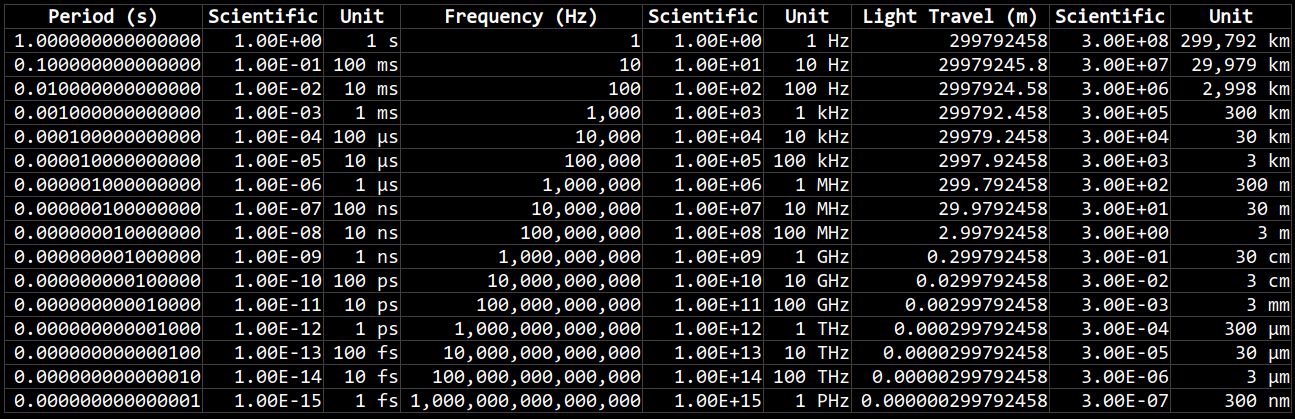# All You Need To Know About ESR METER

Enjoyed watching this one: All You Need To Know About ESR METER To Fix Stuff. How To Use Test Capacitors Inductors Short Finder.

Mentioned were:

ESR stands for Equivalent Series Resistance. You can measure it for capacitors and inductors.

In the video the presenter makes this note: capacitors with these symptoms: double rated capacitance and low ESR, will exhibit very high DC leakage current. He says you should always measure capacitance and ESR when testing caps.

The formula for measuring microhenrys with a 100 kHz ESR meter is: R = 0.628 L; where R is in Ohms (Ω) and L is in microhenrys (µH). So if we measure the ESR we can calculate the inductance.

In the back end of the video he explains how to use an ESR meter to find shorted out MOSFETs in the power stages on a motherboard.

# The DANGERS of Inductor Back EMF

Watched this one today: EEVblog 1409 – The DANGERS of Inductor Back EMF.

I learned about back EMF, freewheeling, snubber, flyback, or clamping diodes…

In this video I also learned that BPJ transistors have a storage time, or switch off time, in the order of microseconds, e.g. 5 μs. That probably explains what I saw when I tried to measure the frequency response of my DTL OR GATE.

# Ground Channel Coupling

Over here I asked about ground channel coupling because I didn’t understand how it could be useful.

All it does is tie the channel input to ground. But it doesn’t do that by shorting the channel input, rather it just substitutes the probe input for a ground input.

Apparently this used to be useful for calibrating old CRO scopes. You would set to ground channel coupling, set ground where you would like it on your cathode ray tube, then set back to either DC or AC coupling.

# Measuring Input Impedance of MSO5074

Over here I asked about the measurements I was getting when I tried to measure the input impedance of my scope with my multimeter.

I was getting a reading I didn’t expect or understand, but that turned out to be because of a dud BNC to banana adapter that I was using.

For some reason this adapter had either a 1.5 MΩ or a 6 MΩ resistance across it. It would change from time to time when I plugged in banana plugs. Weird. I can only assume some sort of manufacturing problem.

I threw the adapter in the bin and measured again with functional equipment and got the reading of 1 MΩ that I was expecting.

# Period and Frequency

Oh, wait. I made it better.These charts show how period, frequency, and the speed of light are related to each other, in scientific and compact units.

Period is measured in seconds, frequency is measures in Hertz (cycles per second), and the distance of light travelled during one period is measured in meters (it is also known as the wavelength). The equation for wavelength is:

λ = c / ν

Where λ is the wavelength in meters, c is the speed of light in meters per second, and ν is the frequency of the wave in Hertz (cycles per second).

Wavelengths between 400 nanometers (nm) for violet light to 700 nm for red light are the visible component of the electromagnetic spectrum. The frequency of red light, on the low end of the visible spectrum, is about 430 terahertz (THz); whereas violet light, on the high end of the visible spectrum, is around 750 THz.